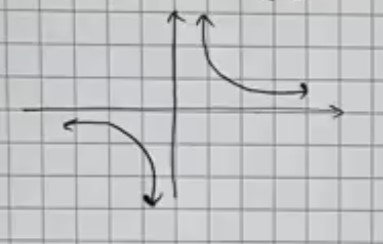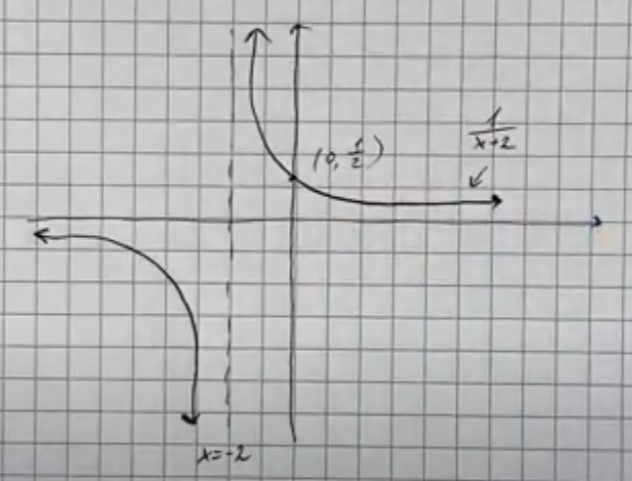# Sketch the following functions using graph transformations: f(x)=1/(x+2)Frederick Kramer 2022-07-15 Answered
Sketch the following functions using graph transformations:
$f\left(x\right)=\frac{1}{x+2}$
You can still ask an expert for help

• Live experts 24/7
• Questions are typically answered in as fast as 30 minutes
• Personalized clear answers

Solve your problem for the price of one coffee

• Math expert for every subject
• Pay only if we can solve itBrendan Bush
$f\left(x\right)=\frac{1}{x+2}$We have step-by-step solutions for your answer!Jeffrey Jordon

Answer is given below (on video)

We have step-by-step solutions for your answer!